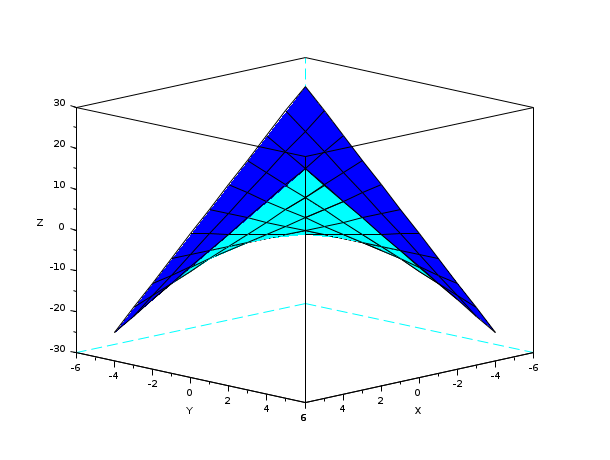Scilab Home page | Wiki | Bug tracker | Forge | Mailing list archives | ATOMS | File exchange
Change language to: English - Français - Português - 日本語 -

Справка Scilab >> Графики > 3d_plot > eval3d

# eval3d

values of a function on a grid

### Calling Sequence

`[z]=eval3d(fun,x,[y])`

### Arguments

fun

function accepting vectors as arguments.

x,y

2 vectors of size (1,n1) and (1,n2). (default value for `y` : `y=x`).

z

matrix of size (n1,n2).

### Description

This function returns a matrix `z(n1,n2)`. `z(i,j)=fun(x(i),y(j))`. If the function `fun` does not accept arguments of type vector use the primitive `feval`.

### Examples

```x=-5:5;y=x;
deff('[z]=f(x,y)',['z= x.*y']);
z=eval3d(f,x,y);
plot3d(x,y,z);

deff('[z]=f(x,y)',['z= x*y']);
z=feval(x,y,f);
plot3d(x,y,z);```